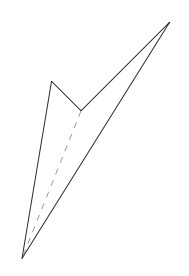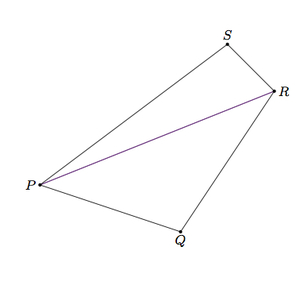##### Engage your students with effective distance learning resources. ACCESS RESOURCES>>

Alignments to Content Standards: G-SRT.A.2

Quadrilaterals $ABCD$ and $EFGH$ have three corresponding congruent angles:

• $m(\angle A) = m(\angle E)$
• $m(\angle B) = m(\angle F)$
• $m(\angle C) = m(\angle G)$
1. Must it be true that $m(\angle D) = m(\angle H)$? Explain.
2. If $ABCD$ and $EFGH$ are isosceles trapezoids, are they always similar?
3. If $ABCD$ and $EFGH$ are rhombuses, are they always similar?

## IM Commentary

The goal of this task is to study if the analogue of the AA criterion for similarity of triangles holds for different types of quadrilaterals. An isosceles trapezoid is a trapezoid with a line of symmetry (see http://en.wikipedia.org/wiki/Isosceles_trapezoid) though they are also sometimes called regular trapezoids. A square and a rectangle which is not a square are perhaps the simplest example of two quadrilaterals sharing three (hence 4) pairs of congruent angles but which are not similar. It is interesting, however, that for certain types of quadrilaterals, such as rhombuses (and hence squares) there is an AAA criterion for similarity. Another interesting question teachers might pose along these lines is: if $ABCD$ is a rhombus (or regular trapezoid), is $EFGH$ necessarily a rhombus (or regular trapezoid)? The answer to both questions is no.

The answer to part (a) is yes even when for quadrilaterals which are not convex. This is because any quadrilateral can be divided into two triangles, each of whose angles sum to 180$^\circ$. An example is pictured below:## Solution

1. If we can show that the sum of the four angles in a quadrilateral are 360$^\circ$ then it follows that $m(\angle D) = m(\angle H)$:

\begin{align} m(\angle D) &= 360 - (m(\angle A) + m(\angle B) + m(\angle C)) \\ &= 360 - (m(\angle E) + m(\angle F) + m(\angle G)) \\ &= m(\angle H). \end{align}

To see why the sum of the angles in a quadrilateral are always 360$^\circ$, we draw in one of the diagonals of the quadrilateral:The sum of the angles in triangle $PQR$ is $180$ degrees and the sum of the angles in triangle $PRS$ is $180$ degrees:

\begin{align} m(\angle RPQ) + m(\angle Q) + m(\angle PRQ) &= 180 \\ m(\angle RPS) + m(\angle S) + m(\angle PRS) &= 180. \end{align}

We have $m(\angle RPQ) + m(\angle RPS) = m(\angle P)$ and $m(\angle PRQ) + m(\angle PRS) = m(\angle R)$ so adding the above two equations gives $$m(\angle P) + m(\angle Q) + m(\angle R) + m(\angle S) = 180 + 180 = 360.$$

2. All regular trapezoids are not similar. Perhaps the simplest case to see this is the special case of rectangles. All rectangles share four congruent right angles but they are not all similar. For example, if $ABCD$ is a 1 by 2 rectangle and $EFGH$ is a 1 by 4 rectangle then they are not similar since there is no common scale factor for the different sides of the rectangles. The problem here is that we have scaled the rectangles in one direction only whereas a dilation of the plane scales in all directions.

This idea can be applied to regular trapezoids which are not rectangles, the idea being that we can simultaneously lengthen the two parallel sides of the trapezoids without changing the angles. So we can take $ABCD$ and $EFGH$ to be regular trapezoids with a pair of 45 degree angles and a pair of 135 degree angles. The side lengths of $ABCD$ can be 1, 2, 2, and 3 (with the parallel sides having lengths 1 and 3). For $EFGH$ we can lengthen the two parallel sides so its side lengths can be 4, 2, 2, and 6. Although they share four congruent angles, these trapezoids are not similar as there is no scale factor which will take side lengths of $ABCD$ to the (corresponding) side lengths of $EFGH$.

3. In this case, $ABCD$ and $EFGH$ are similar. The diagonals $\overline{AC}$ and $\overline{EG}$ bisect congruent angles $A$, $C$, $E$, and $G$ (here we use the fact that opposite angles in a rhombus are congruent). Triangles $ABC$ and $EFG$ share three congruent angles and are therefore similar. Therefore there is a sequence of dilations, translations, rotations, and reflections which maps $\triangle ABC$ to $\triangle EFG$. This sequence of rigid motions will also map $D$ to $H$ because $\triangle ADC$ is the only triangle distinct from $\triangle ABC$, congruent to $\triangle ABC$, sharing side $\overline{AC}$: after the sequence of dilations, translations, rotations, and reflections $\triangle ADC$ maps to the unique triangle distinct from but congruent to $\triangle EFG$ sharing side $EG$, namely $\triangle EHG$.